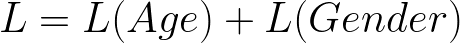by Cole Murray

How to build an age and gender multi-task predictor with deep learning in TensorFlow

In my last tutorial, you learned about how to combine a convolutional neural network and Long short-term memory (LTSM) to create captions given an image. In this tutorial, you’ll learn how to build and train a multi-task machine learning model to predict the age and gender of a subject in an image.

Overview

• Introduction to age and gender model
• Building a Multi-task Tensorflow Estimator
• Training

Prerequisites

• basic understanding of convolutional neural networks (CNN)
• basic understanding of TensorFlow
• GPU (optional)

Introduction to Age and Gender Model

In 2015, researchers from Computer Vision Lab, D-ITET, published a paper DEX and made public their IMDB-WIKI consisting of 500K+ face images with age and gender labels.

DEX outlines an neural network architecture involving a pretrained imagenet vgg16 model that estimates the apparent age in face images. DEX placed first in ChaLearn LAP 2015 — a competition that deals with recognizing people in an image — outperforming human reference.

Age as a classification problem

A conventional way of tackling an age estimation problem with an image as input would be using a regression-based model with mean-squared error as the loss function. DEX models this problem as a classification task, using a softmax classifier with each age represented as a unique class ranging from 1 to 101 and cross-entropy as the loss function.

Multi-task learning is a technique of training on multiple tasks through a shared architecture. Layers at the beginning of the network will learn a joint generalized representation, preventing overfitting to a specific task that may contain noise.

By training with a multi-task network, the network can be trained in parallel on both tasks. This reduces the infrastructure complexity to only one training pipeline. Additionally, the computation required for training is reduced as both tasks are trained simultaneously.

Building a multi-task network in TensorFlow

Below you’ll use TensorFlow’s estimator abstraction to create the model. The model will be trained from raw image input to predict the age and gender of the face image.

Project Structure

.├── Dockerfile├── age_gender_estimation_tutorial│   ├── cnn_estimator.py│   ├── cnn_model.py│   └── dataset.py├── bin│   ├── download-imdb.sh│   ├── predict.py│   ├── preprocess_imdb.py│   └── train.py├── requirements.txt

Environment

For the environment, you’ll use Docker to install dependencies. A GPU version is also provided for convenience.

docker build -t colemurray/age-gender-estimation-tutorial -f Dockerfile .

Data

To train this model, you’ll use the IMDB-WIKI dataset, consisting of 500K+ images. For simplicity, you’ll download the pre-cropped imdb images (7GB). Run the script below to download the data.

Preprocessing

You’ll now process the dataset to clean out low-quality images and crop the input to a fixed image size. Additionally, you’ll format the data as a CSV to simplify reading into TensorFlow.

docker run -v \$PWD:/opt/app \-e PYTHONPATH=\$PYTHONPATH:/opt/app \-it colemurray/age-gender-estimation-tutorial \python3 /opt/app/bin/preprocess_imdb.py \--db-path /opt/app/data/imdb_crop/imdb.mat \--photo-dir /opt/app/data/imdb_crop \--output-dir /opt/app/var \--min-score 1.0 \--img-size 224

After approximately 20 minutes, you’ll have a processed dataset.

Next, you’ll use TensorFlow’s data pipeline module tf.data to provide data to the estimator. Tf.data is an abstraction to read and manipulate a dataset in parallel, utilizing C++ threads for performance.

Here, you’ll utilize TensorFlow’s CSV Reader to parse the data, preprocess the images, create batches, and shuffle.

Model

Below, you’ll create a basic CNN model. The model consists of three convolutions and two fully connected layers, with a softmax classifier head for each task.

Joint loss function

For the training operation, you’ll use the Adam Optimizer. For a loss function, you’ll average the cross-entropy error of each head, creating a shared loss function between the heads.age and gender joint loss function

TensorFlow estimator

TensorFlow estimators provide a simple abstraction for graph creation and runtime processing. TensorFlow has specified an interface model_fn, that can be used to create custom estimators.

Below, you’ll take the network created above and create training, eval, and predict. These specifications will be used by TensorFlow’s estimator class to alter the behavior of the graph.

Train

Now that you’ve preprocessed the data and created the model architecture and data pipeline, you’ll begin training the model.

docker run -v \$PWD:/opt/app \-e PYTHONPATH=\$PYTHONPATH:/opt/app \-it colemurray/age-gender-estimation-tutorial:gpu \python3 /opt/app/bin/train.py \--img-dir /opt/app/var/crop \--train-csv /opt/app/var/train.csv \--val-csv /opt/app/var/val.csv \--model-dir /opt/app/var/cnn-model \--img-size 224 \--num-steps 200000

Predict

Below, you’ll load your age and gender TensorFlow model. The model will be loaded from disk and predict on the provided image.

# Update the model path below with your modeldocker run -v \$PWD:/opt/app \-e PYTHONPATH=\$PYTHONPATH:/opt/app \-it colemurray/age-gender-estimation-tutorial \python3 /opt/app/bin/predict.py \--image-path /opt/app/var/crop/25/nm0000325_rm2755562752_1956-1-7_2002.jpg \--model-dir /opt/app/var/cnn-model-3/serving/<TIMESTAMP>

Conclusion

In this tutorial, you learned how to build and train a multi-task network for predicting a subject’s age and image. By using a shared architecture, both targets can be trained and predicted simultaneously.

Next Steps:

• Evaluate on Your Own Dataset
• Try a different network architecture
• Experiment with Different Hyperparameters

Questions/issues? Open an issue here on GitHub

Complete code here.

Call to Action

If you enjoyed this tutorial, follow and recommend!

Interested in learning more about Deep Learning / Machine Learning? Check out my other tutorials:

- Building an image caption generator with Deep Learning in Tensorflow

- Building a Facial Recognition Pipeline with Deep Learning in Tensorflow

- Deep Learning CNN’s in Tensorflow with GPUs

- Deep Learning with Keras on Google Compute Engine

- Recommendation Systems with Apache Spark on Google Compute Engine

Other places you can find me: